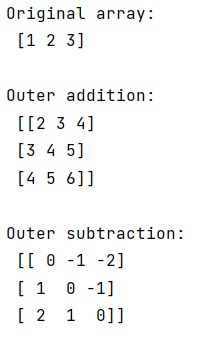# How to perform outer addition with NumPy?

Learn, how can we perform outer addition with Python NumPy?
Submitted by Pranit Sharma, on March 07, 2023

## Performing outer addition with NumPy

Suppose that we are given a numpy array and we need to perform outer addition/multiplication using some technique rather than using a for loop.

Many of NumPy's basic operators such as numpy.add(), numpy.subtract(), numpy.multiply(), etc. are known as universal functions (ufuncs)

In numpy, all universal functions that take two input arguments have an attribute called outer. With the help of this attribute, all the outer entities can be used whether in addition, subtraction, or multiplication.

Let us understand with the help of an example,

## Python code to perform outer addition with NumPy

```# Import numpy
import numpy as np

# Creating array
arr = np.array([1, 2, 3])

# Display Original array
print("Original array:\n",arr,"\n")

# Display result

# Outer subtraction
res = np.subtract.outer(arr,arr)

# Display result
print("Outer subtraction:\n",res,"\n")
```

Output:Languages: » C » C++ » C++ STL » Java » Data Structure » C#.Net » Android » Kotlin » SQL
Web Technologies: » PHP » Python » JavaScript » CSS » Ajax » Node.js » Web programming/HTML
Solved programs: » C » C++ » DS » Java » C#
Aptitude que. & ans.: » C » C++ » Java » DBMS
Interview que. & ans.: » C » Embedded C » Java » SEO » HR
CS Subjects: » CS Basics » O.S. » Networks » DBMS » Embedded Systems » Cloud Computing
» Machine learning » CS Organizations » Linux » DOS
More: » Articles » Puzzles » News/Updates